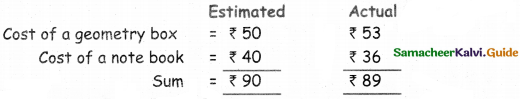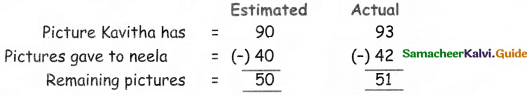Students can download 5th Maths Term 3 Chapter 2 Numbers Ex 2.1 Questions and Answers, Notes, Samacheer Kalvi 5th Maths Guide Pdf helps you to revise the complete Tamilnadu State Board New Syllabus, helps students complete homework assignments and to score high marks in board exams.

## Tamilnadu Samacheer Kalvi 5th Maths Solutions Term 3 Chapter 2 Numbers Ex 2.1

Question 1.
Fill in the blanks

(i) Estimation of 27 to its nearest Tens place is ———–
30

(ii) Estimation of 65 to its nearest Tens place is ———–
70(iii) The cost of 1kg pomegranate is? 93. Then the estimated cost is
90

(iv) Estimation of 76 bananas to its nearest Tens place is ———–
80

Question 2.
There are 27 girls and 38 boys in a class. Find the sum and estimate the sum to its nearest Tens place.
Ans:
Number of girls = 30
Number of boys = 40
Sum = 30 + 40
= 70Question 3.
If the cost of a geometry box is ₹ 53 and the cost of a note book is ₹ 36, then estimate their cost to its nearest Tens place and find the sum. Also, find the difference between the estimated value and the actual value.Question 4.
If Kavitha has 93 pictures and she gave 42 pictures to her friend Neela, then estimate the picutres to its nearest Tens place and find their difference. Also, find the difference between actual value and the estimated value,Question 5.
The cost of a pen is ₹ 32. Find the cost of 6 pens and estimate it to its nearest Tens place.
Cost of a pen = ₹ 32
Cost of 5 pens = 32 × 6 = 192.Question 6.
Arun has ₹ 47, Raja has nearest Hundreds place. ₹ 54. Find the sum and estimate it to its nearest Hundreds place.
Money A run has = Rs. 47
Money Raja has = Rs. 54
Sum = Rs. 47 + Rs 54 = 101
Sum ~ 100

Question 7.
There are 21 chocolate bars in a packet. Find the number of chocolate bars in 9 such packets and estimate it to its nearest Hundreds place.